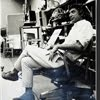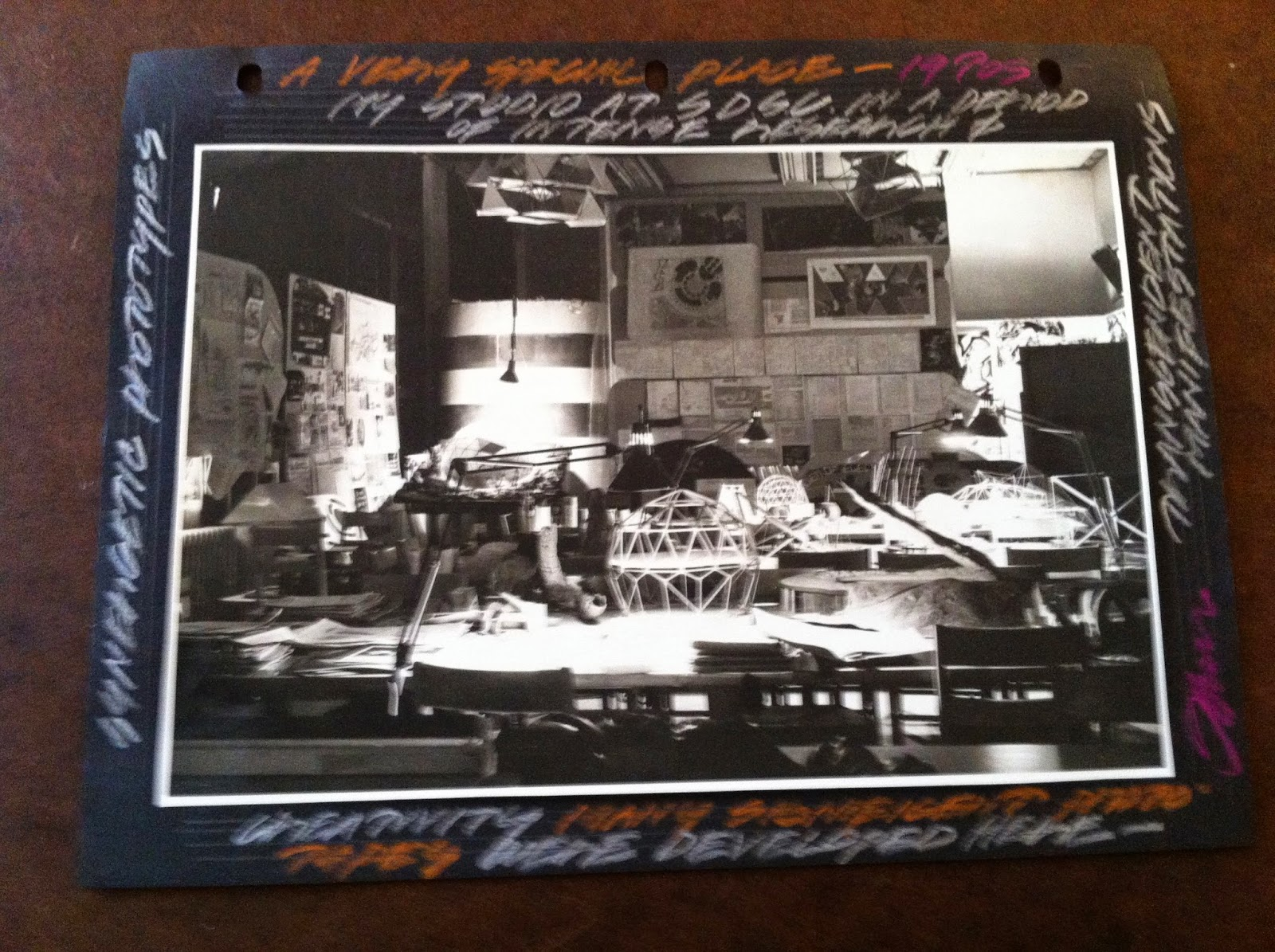## Eugene Ray SDSU 1970'sEugene Ray 1970's. photo credit: Tom Davis

## Saturday, May 31, 2014

### FLASH OF LIGHT / evening sky

+ARCHITECTURE IS NOT JUST MATTER+
>it is more importantly what you don't see that is<
>an essence that makes architecture so important<
+FULCANELLI+
>and other enlightened egyptian alchemist seers<
>(papus & peladan) at the marvelous bookshop<
^^^^^^^^^^^^^^^^^^^^^^^^^^^^^^
+GOOGLE : EUGENE RAY / DEGRUY/
+BOOKSHOP OF THE MARVELOUS+
^^^^^^^^^^^^^^^^^^^^^^^^^^^
>bookshops in paris in the 19th century<
>were magnificent gathering places for<
>scholars of alchemy who were great<
+ARTISTS & MAGICIANS /+
+ST SULPICE SCHOLARS+
>were on the cutting edge of a creative<
>exploration for an enlightened world<
^^^^^^^^^^^^^^^^^^^^^^
>notre dame cathedral (on the cover here)<
>was a gothic geomancy st bernard vision<
+(CORBU was not CATHOLIC,+
>that inspired scholars at st sulpice, such<
>as pere couturier corbu's great patron<
^^^^^^^^^^^^^^^^^^^^^
>stonehenge is one of those ancient cosmic<
>inspired sites that illuminated st bernard<
+(ET CROP CIRCLES+
+SEEN HERE (many)) !
>and his pere couturier scholar corbu<
^^^^^^^^^^^^^^^^^^^^^^
>de ray castle on the saone river in burgundy<
>is where the turin shroud was first brought<
+/ DEGRUY / de RAY CASTLE)+
>there were 13 original towers in the 12th C<
^^^^^^^^^^^^^^^^^^^^^^^^^^
>in 1965 this, probably the best biomorphic<
>design, i have ever made flowed from my<
+(COSMIC BIOTRONICS via+
+COMPOUND CURVES)+ !
>pencil as if by a stroke of synchronicity<
^^^^^^^^^^^^^^^^^^^^^^^
>four years later, by 1969 i had completed<
>four projects in new orleans of color, light<
+COLOR-LIGHT- ENERGY+
>and gathered my family to head to sdsu to<
> do my best to share the vision w/ others<
^^^^^^^^^^^^^^^^^^^^^^^^^^^
>(announcement for my retrospective<
>of 1980 at san diego state university)<
^^^^^^^^^^^^^^^^^^^^^^^^
>synchronistically by 1980 i had completed<
>"silver ship" and constructed an exhibition<
+10 YEARS IN CALIFORNIA)+
>of all the work in california for 10 years<
^^^^^^^^^^^^^^^^^^^^^^^^^^
>synchronistically "silver ship" was built on<
>nautilus st. (perfection) in lajolla california<
^^^^^^^^^^^^^^^^^^^^^^^^^
>the technology of ufo craft is mind boggling<
>only a study of our natural elements can be<
+(COMPOUND CURVED+
+UFOs PLUS SHELLs+
+ARE VERY STRONG)+ !
>comparative to the ufo structural perfection<
^^^^^^^^^^^^^^^^^^^^^^^^^^
>only leonardo da vinci was advanced enough<
>in the 16th century to design this fortress for<
+(COMPOUND CURVE WALLS+
+PROVIDE MUCH STRENGTH)+
>"valentine" borgia, francois I brother-in-law<
^^^^^^^^^^^^^^^^^^^^^^^^^^^^
>the dna memory phenomena is fascinating<
>and constantly links my focus with the<
+TO ST. BERNARD VISION+
>ray-bresse-gruy-savoy-vergy-charney<
^^^^^^^^^^^^^^^^^^^^^^^^>our sdsu classroom-studios were settings<
>for much technical vision & exploration<
^^^^^^^^^^^^^^^^^^^^^^^^^>little did i realize at the time how important<
>our effort at san diego state, tulane & lsu<
+(OUR MESSAGE IS NOW+
+SEEN DAILY in EUROPE)+ !
>would ultimately be via the world wide<
>link of this website to many countries<
^^^^^^^^^^^^^^^^^^^^^^^
>kerlian photography has revealed the energy<
>emitted from pyramids in several countries<
^^^^^^^^^^^^^^^^^^^^^^^^^
>tonight's blog ends w/ an image from the<
>crash at roswell on the cover of dr. bob<
>and ryan woods ufo crash conf. book<
+(1947 WAS the YEAR of MY+
+SIGHTING plus ROSWELL)+
>next to a page from ron edens book<
>devoted to my life & work which<
>provides a very interesting sync<
^^^^^^^^^^^^^^^^^^^^^
+GEORGE NOORY BELIEVES+
+there are NO COINCIDENCES+ !
^^^^^^^^^^^^^^^^^^^^^^
eugene ray, mfa, architect
professor emeritus, sdsu

## Friday, May 30, 2014

+CORBU IS IN MY BLOOD+
>google : eugene ray / lecorbusier<
^^^^^^^^^^^^^^^^^^^^^
+CORBU'S TEMPLAR-MASON+
>(swiss/french gruy similarity)<
+INFLUENCE WAS STRONG+
^^^^^^^^^^^^^^^^^^^^
>his affinity w/ the geomancy of saint<
>bernard in the gruy orient forest is+
+VERY IMPRESSIVE+ !
>(corbu studied bernard's abbeys
>when he designed "la tourette")
^^^^^^^^^^^^^^^^
+"THE MODULAR"+
>good easy, & the bad, difficult"<
^^^^^^^^^^^^^^^^^^^^^
>corbu painted in the morning while<
+(PERE COUTURIER+
+/ (GRUY) PATRON)+
>designing buildings in afternoons<
^^^^^^^^^^^^^^^^^^^^^^^
>light was important in corbu's early<
+(LIGHT=HEALTH /was+
+BUNGALOW VISION)+
>house built for his wizened mother<
^^^^^^^^^^^^^^^^^^^^
>corbu built a small house for his mother<
+SAVOY-BRESSE-GRUY)+
>near gruy castles just above lake geneva<
^^^^^^^^^^^^^^^^^^^^^^^
>corbu's paris studio was in a jesuit urban<
+/CORBU in PARIS,(images)+
>setting/ (35 rue dr sevres, paris corridor)<
^^^^^^^^^^^^^^^^^^^^^^^^^^
>einstein was corbu's brilliant friend and<
+(COSMIC CONSCIOUS+
+VISION WAS VITAL)<
^^^^^^^^^^^^^^^^^^^^^^^^
>andre malreaux, corbu's great friend was the<
+(RENE de ANJOU / JOAN d'ARC+
+/flew TEMPLAR CROSS FLAG)+
>minister of culture who flew a templar flag<
>w/ charles de gaulle during the 2nd w.w.<
^^^^^^^^^^^^^^^^^^^^^^^^

>corbu's mother was from "le chaux de fonds"<
+de RAY CASTLE, (TEMPLAR+
+SHROUD IMPORT) is NEAR+
>+templar/freemason+swiss-french village<
^^^^^^^^^^^^^^^^^^^^^^^^^^^^
>corbu documentation of the construction<
+(I SAW the ROOF "CHILD'S+
+ZONE" IN SUMMER OF 69)
>of the "unite de habitation" in marseilles<
^^^^^^^^^^^^^^^^^^^^^^^^^
>this magnificent tensile structure was<
+"THE ELECTRONIC POEM"+
+(2nd ONLY to RONCHAMP)+
>built for the worlds fair in brussels<
^^^^^^^^^^^^^^^^^^^^^^^^

>giant slide projections on the interior<
+(JESUS was a SYMBOL+
+/of TEMPLAR VISION)+
>of the "electronic poem" in brussels<
^^^^^^^^^^^^^^^^^^^^^^^
>note : i am grateful to google for collecting<
>my : "eugene ray-corbu in paris" images<
^^^^^^^^^^^^^^^^^^^^^^^^^
+(GET THE TABLE FOR+
+JEAN PAUL SARTRE+
>when in paris dine @ "garden of lilacs")<
^^^^^^^^^^^^^^^^^^^^^^^^^^^
>it is important to know that the world's<
>most important designs came from a<
+(CORBU=VISIONARY+
+MONK & MAGICIAN)+
>former paris jesuit abbey corridor<
^^^^^^^^^^^^^^^^^^^^^^^

+CORBU : PAINTER of the ORGANIC+
>organic fertility going back<
>deep into the orient forest<
>(scholar of african culture)<
^^^^^^^^^^^^^^^^^^^^^^^^
eugene ray, mfa, architect
professor emeritus, sdsu

## Thursday, May 29, 2014

>was the primary subject of research in all<
+/ KING RENE de ANJOU+
+(FOCUS ON "IMAGES")+
>of the great libraries of early civilization<
^^^^^^^^^^^^^^^^^^^^^^^^
+ARTISTS=POETS=SEERS+
>are vivid practitioners of the ancient<
>ability to synergize ingredients to<
>create transcendent manifestation<
^^^^^^^^^^^^^^^^^^^
+MANLY PALMER HALL+
>(our focus on this night<
>w/ a. & a. le plongeon)<
+SCHOLAR of ALCHEMY+
^^^^^^^^^^^^^^^^
>godard and his french "new wave" cinema<
>colleagues from the sixties were masters<
+(>"BREATHLESS"< WAS A+
+GODARD STAR CINEMA)+
>of the alchemy of cinematic assemblage<
^^^^^^^^^^^^^^^^^^^^^^^^^^^
>jean luc godard has the talents to assemble<
>cinematic images to create magical artistry<
+(COSMIC CONSCIOUSNESS+
+WOULD AID HIS VISION)+
>he deserves to win the cannes"palm d'or"<
^^^^^^^^^^^^^^^^^^^^^^^^^^
>anthony bourdain's gastronomic tv programs<
>places him w/ many international alchemists<
+(EXOTIC INGREDIANTS+
+ORGANIC SYNERGY)+
>from the far corners of radiant planet earth<
^^^^^^^^^^^^^^^^^^^^^^^^^^
+GOOGLE : EUGENE RAY / ALCHEMY+
>(to find my oeuvre of healing prototypes<
>result of our cosmic conscious research)<
^^^^^^^^^^^^^^^^^^^^^^^^^
>part of our quest in los angeles at the getty<
>center was the archive of manly p. hall a<
+(MANLY PALMER HALL+
+MASTER OF ALCHEMY)+
>gifted scholar of the ancient alchemists<
^^^^^^^^^^^^^^^^^^^^^^^^
>victor hugo, cousin of francois verloin degruy's<
>wife and his contemporary friend in paris was<
+(HUGO & CHARLES NODIER /+
+SION PRIORY, GRUY VISION)+
>a devoted scholar of french 19th C alchemy<
^^^^^^^^^^^^^^^^^^^^^^^^^^^^
>profound artistry is produced after research<
+(METAPHYSICAL ART+
+our GREAT REWARD)+
>reveals ancient secrets with cosmic vision<
^^^^^^^^^^^^^^^^^^^^^^^^^
>alchemy has meant much to my life and work<
>especially last five years in new orleans while<
+(COLOR-LIGHT-ENERGY+
>building 4 projects (tulane university above)<
^^^^^^^^^^^^^^^^^^^^^^^^^
>the tetrahedral biotronic energy seen here<
>via kerlian photography is the perfect use<
+(GOOD HEALTH SPA'S+
>of the alchemy of radiant force fields<
^^^^^^^^^^^^^^^^^^^^^^^

>pythagoras of crotona, transcendent alchemist<
>of mathematics, music & astronomy as seen<
+THE GREAT ALCHEMIST)+
>in this symbolic portrait c/o manly p. hall<
^^^^^^^^^^^^^^^^^^^^^^^^^^
>the pythagorean signet ring with its five point<
>pentagram was a highly regarded talisman of<
+(TALISMAN OF THE GRUY+
+IN ORIENTAL FOREST, FR)+
>good health by the ancient orient alchemists<
^^^^^^^^^^^^^^^^^^^^^^^^^^

>magician-alchemist of the tabernacle at work<
>in the orient was inspiration for the ancient<
+(GEOMANCY WAS VITAL+
+COSMIC SCHOLARSHIP)+
>crusaders from gruyere's and orient forest<
^^^^^^^^^^^^^^^^^^^^^^^^^^
+GETTY CENTER PURCHASED THE<
+HUGE ARCHIVE OF MANLY HALL+
>(getty shall mount a profound<
>exhibition in the near future<
>archive holdings are on-line)<
+PLUS AUGUSTUS le PLONGEON+
+& ALICE DIXON le PLONGEON+
^^^^^^^^^^^^^^^^^^^^^^^^^^
eugene ray, mfa, architect
professor emeritus, sdsu

## Wednesday, May 28, 2014

### LIFE AS RADIANT CINEMA /

+DOCUMENTING LIFE & WORK+
>(inspired by lecorbusier, goff<
>wright, gaudi, fuller, etc)<
+VIA THE ART OF COLLAGE+
^^^^^^^^^^^^^^^^^^^^^^
>since about year 1946 i have photographed<
>my life and work with a series of cameras<
+(MERCURY to LEICA+
+35MM CAMERAS)+
>here is a selection of the docu-photos<
^^^^^^^^^^^^^^^^^^^^^^^^^^
+FRIENDS ARE A VITAL PART+
>(family and friends are the<
>soul of our path in life)<
+OF LIFE DOCUMENTATION+
^^^^^^^^^^^^^^^^^^^^^^
>christopher friedrichs, new orleans landscape<
+(CHRIS WAS FAMED for+
+HIS NOLA GARDENS)+
>architect was my friend and LSU classmate<
>(four images / docu-collage page concept)<
^^^^^^^^^^^^^^^^^^^^^^^^^^
>french organic syndrome expressed with a<
>torn new orleans french baguette wrapper<
+(WORDS & PHOTOGRAPHS+
+A POWERFUL SYNERGY)+
^^^^^^^^^^^^^^^^^^^^^^^^
>french creole tombs and country churches<
>create a b&w background for radiant calif<
+FROM THE SOIL OF DECAY)+
>architecture and synergetic biomorphics<
^^^^^^^^^^^^^^^^^^^^^^^
>a+u publication, japan (right)<
^^^^^^^^^^^^^^^^^^^
>a+u publication, japan, 1985<
^^^^^^^^^^^^^^^^^^^
>in 1985 my architecture and photo inventions<
>were published and exhibited in france and<
+(FRANCE & JAPAN, PUB 1985+
>japan by A+U and the paris biennale<
^^^^^^^^^^^^^^^^^^^^^^^^^
>synergetic environs, 4 image photo collage<
^^^^^^^^^^^^^^^^^^^^^^^
>biotronic energy structures became my major<
>focus and photo-collage an increasingly vital<
+(SDSU SYNERGETICS TO+
+LECTURES IN LONDON)+
>asset in communicating the radiant concepts<
^^^^^^^^^^^^^^^^^^^^^^^^^
>thousands of photos, many yet unseen<
>are in my lecture cylinders given sdsu<
^^^^^^^^^^^^^^^^^^^^^^^
>color, light energy in new orleans to the<
>biotronic structures in california photo<
>techniques became an important tool<
^^^^^^^^^^^^^^^^^^^^^
>our first sdsu arizona field trip to wright,<
>soleri structures, (tom davis included)<
^^^^^^^^^^^^^^^^^^^^^
>field trips, prototypes and exhibitions were the<
>subject of much photo-collage xerography and<
+(XEROGRAPHY WAS EFFICIENT+
+AFFORDABLE & AESTHETIC)+
>correspondence art the communication tool<
^^^^^^^^^^^^^^^^^^^^^^^^^^^^
>wilty residence, (times picayune roto)<
>typical mail art color xerox document<
^^^^^^^^^^^^^^^^^^^^^^^
>my oeuvre had a balanced content of built<
>structures w/ research oriented prototypes<
+new orleans TROPIC DESIGN)+
>creativity & invention always important<
^^^^^^^^^^^^^^^^^^^^^^^^
>the plant kingdom was at the heart of my<
>metaphysical photo collage graphics and<
+/ PLANT KINGDOM+
>these became the soul of mailed packets<
^^^^^^^^^^^^^^^^^^^^^^^^^
>a corner of my studio at the end revealed<
>a plethora of photo-collage documenta &,<